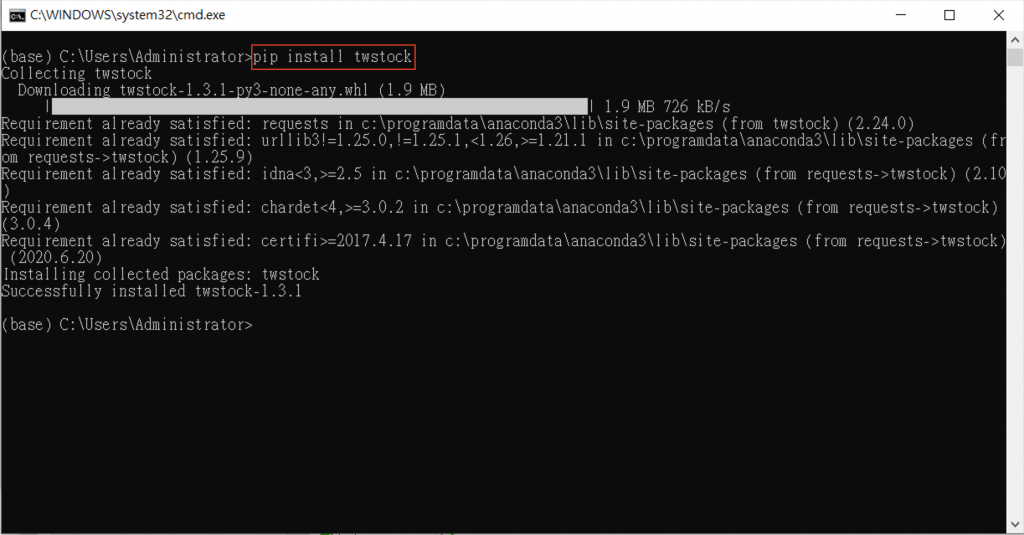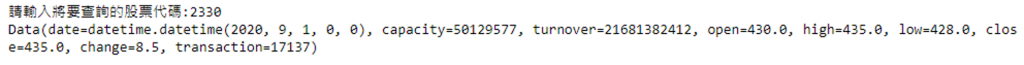#DAY 24
1
Software Development

## [2020鐵人賽Day24]糊裡糊塗Python就上手-數據化圖之術(下)

### Install twstock

twstock 台灣股市股票價格擷取
https://pypi.org/project/twstock/

``````pip install twstock
``````### 實作台股個股股價折線圖

#### 導入將使用的模組

``````import matplotlib.pyplot as plt
import twstock
import datetime
``````

#### 設置詢問使用者股票代碼

``````# 詢問使用者股票代碼
stock_code = input("請輸入將要查詢的股票代碼:")
``````

#### 建立 Stock 物件

``````stock = twstock.Stock(stock_code) # 建立 Stock 物件
``````

#### 取得查詢當年年份及上個月月份

``````now_date = datetime.datetime.now() # 取得查詢當下的時間
now_year = now_date.year # 取得查詢當下當年年份

# 取得查詢當下上個月月份
if(now_date.month != 1):
last_month = now_date.month - 1
else:
last_month = 12
``````

#### 取得「查詢年/查詢時間前一個月」的股票資料

``````stock.fetch(year, month)
``````

``````stocklist = stock.fetch(now_year, last_month)
``````

#### 建立 x, y 軸串列，x 軸為日期時間(date)，y 軸為收盤價(close)

``````print(stock.data)
````````````listx = []
listy = []
for value in stocklist:
listx.append(value.date.strftime('%Y-%m-%d'))
listy.append(value.close)
``````

#### 設定圖表區寬高

``````plt.figure(figsize=(10,10)) # 設定圖表區寬高
``````

#### 設定 x 軸標題、y 軸標題、圖表標題

``````plt.xlabel('日期', fontsize="16") # 設定 x 軸標題內容及大小
plt.ylabel('股價', fontsize="16") # 設定 y 軸標題標題內容及大小
plt.title('Taiwan Stock', fontsize="18") # 設定圖表標題內容及大小
``````

#### 將 x軸串列資料，y軸串列資料，使用 plot 函式將折線圖實現出來，並且顏色為紅色，標記為「點」，標記大小為 16

``````plt.plot(listx, listy, color='red', markersize="16", marker=".") # 紅色，實線，標記大小 16，標記為「點」
``````

#### 讓 x 坐標軸標題旋轉 45 度，使得文字不會重疊

``````plt.xticks(rotation = 45) # 讓 x 坐標軸標題旋轉 45 度
``````

#### 中文亂碼，解決方法

``````# 設定讓中文可順利顯示不亂碼
plt.rcParams["font.sans-serif"] = "Microsoft JhengHei" # 將字體換成 Microsoft JhengHei
``````

``````plt.rcParams["axes.unicode_minus"] = False # 讓負號可正常顯示
``````

#### 將圖表呈現出來

``````plt.show()
``````

### 完整代碼

``````import matplotlib.pyplot as plt
import twstock
import datetime

# 詢問使用者股票代碼
stock_code = input("請輸入將要查詢的股票代碼:")

stock = twstock.Stock(stock_code) # 建立 Stock 物件
now_date = datetime.datetime.now() # 取得查詢當下的時間
now_year = now_date.year # 取得查詢當下當年年份

# 取得查詢當下上個月月份
if(now_date.month != 1):
last_month = now_date.month - 1
else:
last_month = 12

stocklist = stock.fetch(now_year, last_month)

listx = []
listy = []
for value in stocklist:
listx.append(value.date.strftime('%Y-%m-%d'))
listy.append(value.close)

plt.figure(figsize=(10,10)) # 設定圖表區寬高
plt.xlabel('日期', fontsize="16") # 設定 x 軸標題內容及大小
plt.ylabel('股價', fontsize="16") # 設定 y 軸標題標題內容及大小
plt.title('Taiwan Stock', fontsize="18") # 設定圖表標題內容及大小
plt.plot(listx, listy, color='red', markersize="16", marker=".") # 紅色，實線，標記大小 16，標記為「點」
plt.xticks(rotation = 45) # 讓 x 坐標軸標題旋轉 45 度

# 設定讓中文可順利顯示不亂碼
plt.rcParams["font.sans-serif"] = "Microsoft JhengHei" # 將字體換成 Microsoft JhengHei
plt.rcParams["axes.unicode_minus"] = False # 讓負號可正常顯示

plt.show()
``````